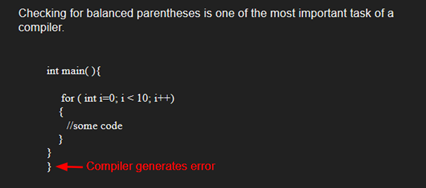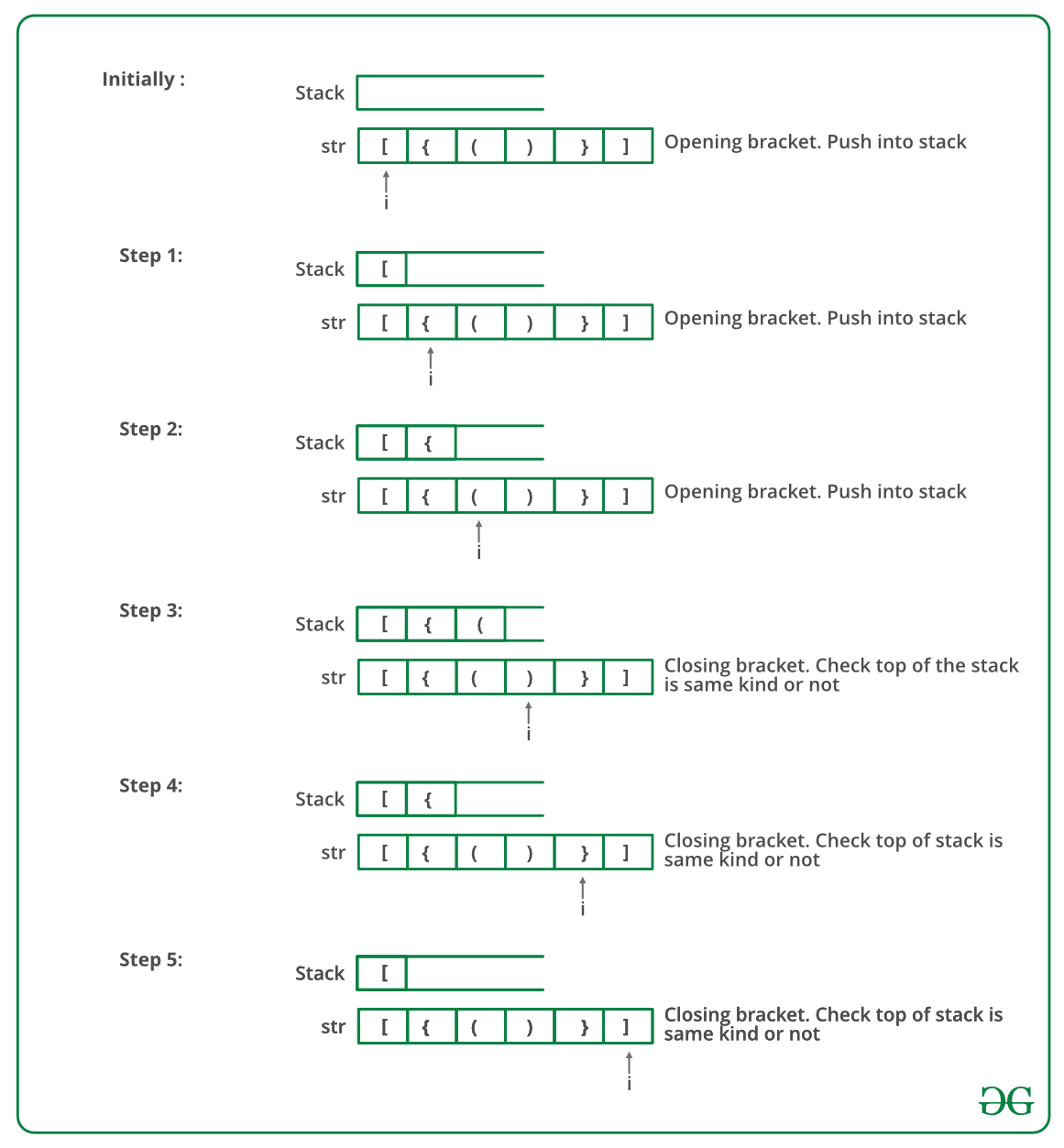# Check for Balanced Brackets in an expression (well-formedness) using Stack

• Difficulty Level : Easy
• Last Updated : 16 Jun, 2022

Given an expression string exp, write a program to examine whether the pairs and the orders of “{“, “}”, “(“, “)”, “[“, “]” are correct in exp.

Example

Input: exp = “[()]{}{[()()]()}”
Output: Balanced

Input: exp = “[(])”
Output: Not BalancedAlgorithm:

• Declare a character stack S.
• Now traverse the expression string exp.
1. If the current character is a starting bracket (‘(‘ or ‘{‘ or ‘[‘) then push it to stack.
2. If the current character is a closing bracket (‘)’ or ‘}’ or ‘]’) then pop from stack and if the popped character is the matching starting bracket then fine else brackets are not balanced.
• After complete traversal, if there is some starting bracket left in stack then “not balanced”

Below image is a dry run of the above approach:Below is the implementation of the above approach:

## C++

 `// CPP program to check for balanced brackets.` `#include ` `using` `namespace` `std;`   `// function to check if brackets are balanced` `bool` `areBracketsBalanced(string expr)` `{  ` `    ``stack<``char``> temp;` `        ``for``(``int` `i=0;i

## C

 `#include ` `#include ` `#define bool int`   `// structure of a stack node` `struct` `sNode {` `    ``char` `data;` `    ``struct` `sNode* next;` `};`   `// Function to push an item to stack` `void` `push(``struct` `sNode** top_ref, ``int` `new_data);`   `// Function to pop an item from stack` `int` `pop(``struct` `sNode** top_ref);`   `// Returns 1 if character1 and character2 are matching left` `// and right Brackets` `bool` `isMatchingPair(``char` `character1, ``char` `character2)` `{` `    ``if` `(character1 == ``'('` `&& character2 == ``')'``)` `        ``return` `1;` `    ``else` `if` `(character1 == ``'{'` `&& character2 == ``'}'``)` `        ``return` `1;` `    ``else` `if` `(character1 == ``'['` `&& character2 == ``']'``)` `        ``return` `1;` `    ``else` `        ``return` `0;` `}`   `// Return 1 if expression has balanced Brackets` `bool` `areBracketsBalanced(``char` `exp``[])` `{` `    ``int` `i = 0;`   `    ``// Declare an empty character stack` `    ``struct` `sNode* stack = NULL;`   `    ``// Traverse the given expression to check matching` `    ``// brackets` `    ``while` `(``exp``[i]) ` `    ``{` `        ``// If the exp[i] is a starting bracket then push` `        ``// it` `        ``if` `(``exp``[i] == ``'{'` `|| ``exp``[i] == ``'('` `|| ``exp``[i] == ``'['``)` `            ``push(&stack, ``exp``[i]);`   `        ``// If exp[i] is an ending bracket then pop from` `        ``// stack and check if the popped bracket is a` `        ``// matching pair*/` `        ``if` `(``exp``[i] == ``'}'` `|| ``exp``[i] == ``')'` `            ``|| ``exp``[i] == ``']'``) {`   `            ``// If we see an ending bracket without a pair` `            ``// then return false` `            ``if` `(stack == NULL)` `                ``return` `0;`   `            ``// Pop the top element from stack, if it is not` `            ``// a pair bracket of character then there is a` `            ``// mismatch.` `            ``// his happens for expressions like {(})` `            ``else` `if` `(!isMatchingPair(pop(&stack), ``exp``[i]))` `                ``return` `0;` `        ``}` `        ``i++;` `    ``}`   `    ``// If there is something left in expression then there` `    ``// is a starting bracket without a closing` `    ``// bracket` `    ``if` `(stack == NULL)` `        ``return` `1; ``// balanced` `    ``else` `        ``return` `0; ``// not balanced` `}`   `// Driver code` `int` `main()` `{` `    ``char` `exp`` = ``"{()}[]"``;`   `    ``// Function call` `    ``if` `(areBracketsBalanced(``exp``))` `        ``printf``(``"Balanced \n"``);` `    ``else` `        ``printf``(``"Not Balanced \n"``);` `    ``return` `0;` `}`   `// Function to push an item to stack` `void` `push(``struct` `sNode** top_ref, ``int` `new_data)` `{` `    ``// allocate node` `    ``struct` `sNode* new_node` `        ``= (``struct` `sNode*)``malloc``(``sizeof``(``struct` `sNode));`   `    ``if` `(new_node == NULL) {` `        ``printf``(``"Stack overflow n"``);` `        ``getchar``();` `        ``exit``(0);` `    ``}`   `    ``// put in the data` `    ``new_node->data = new_data;`   `    ``// link the old list off the new node` `    ``new_node->next = (*top_ref);`   `    ``// move the head to point to the new node` `    ``(*top_ref) = new_node;` `}`   `// Function to pop an item from stack` `int` `pop(``struct` `sNode** top_ref)` `{` `    ``char` `res;` `    ``struct` `sNode* top;`   `    ``// If stack is empty then error` `    ``if` `(*top_ref == NULL) {` `        ``printf``(``"Stack overflow n"``);` `        ``getchar``();` `        ``exit``(0);` `    ``}` `    ``else` `{` `        ``top = *top_ref;` `        ``res = top->data;` `        ``*top_ref = top->next;` `        ``free``(top);` `        ``return` `res;` `    ``}` `}`

## Java

 `// Java program for checking` `// balanced brackets` `import` `java.util.*;`   `public` `class` `BalancedBrackets {`   `    ``// function to check if brackets are balanced` `    ``static` `boolean` `areBracketsBalanced(String expr)` `    ``{` `        ``// Using ArrayDeque is faster than using Stack class` `        ``Deque stack` `            ``= ``new` `ArrayDeque();`   `        ``// Traversing the Expression` `        ``for` `(``int` `i = ``0``; i < expr.length(); i++) ` `        ``{` `            ``char` `x = expr.charAt(i);`   `            ``if` `(x == ``'('` `|| x == ``'['` `|| x == ``'{'``) ` `            ``{` `                ``// Push the element in the stack` `                ``stack.push(x);` `                ``continue``;` `            ``}`   `            ``// If current character is not opening` `            ``// bracket, then it must be closing. So stack` `            ``// cannot be empty at this point.` `            ``if` `(stack.isEmpty())` `                ``return` `false``;` `            ``char` `check;` `            ``switch` `(x) {` `            ``case` `')'``:` `                ``check = stack.pop();` `                ``if` `(check == ``'{'` `|| check == ``'['``)` `                    ``return` `false``;` `                ``break``;`   `            ``case` `'}'``:` `                ``check = stack.pop();` `                ``if` `(check == ``'('` `|| check == ``'['``)` `                    ``return` `false``;` `                ``break``;`   `            ``case` `']'``:` `                ``check = stack.pop();` `                ``if` `(check == ``'('` `|| check == ``'{'``)` `                    ``return` `false``;` `                ``break``;` `            ``}` `        ``}`   `        ``// Check Empty Stack` `        ``return` `(stack.isEmpty());` `    ``}`   `    ``// Driver code` `    ``public` `static` `void` `main(String[] args)` `    ``{` `        ``String expr = ``"([{}])"``;`   `        ``// Function call` `        ``if` `(areBracketsBalanced(expr))` `            ``System.out.println(``"Balanced "``);` `        ``else` `            ``System.out.println(``"Not Balanced "``);` `    ``}` `}`

## Python3

 `# Python3 program to check for` `# balanced brackets.`   `# function to check if` `# brackets are balanced`     `def` `areBracketsBalanced(expr):` `    ``stack ``=` `[]`   `    ``# Traversing the Expression` `    ``for` `char ``in` `expr:` `        ``if` `char ``in` `[``"("``, ``"{"``, ``"["``]:`   `            ``# Push the element in the stack` `            ``stack.append(char)` `        ``else``:`   `            ``# IF current character is not opening` `            ``# bracket, then it must be closing.` `            ``# So stack cannot be empty at this point.` `            ``if` `not` `stack:` `                ``return` `False` `            ``current_char ``=` `stack.pop()` `            ``if` `current_char ``=``=` `'('``:` `                ``if` `char !``=` `")"``:` `                    ``return` `False` `            ``if` `current_char ``=``=` `'{'``:` `                ``if` `char !``=` `"}"``:` `                    ``return` `False` `            ``if` `current_char ``=``=` `'['``:` `                ``if` `char !``=` `"]"``:` `                    ``return` `False`   `    ``# Check Empty Stack` `    ``if` `stack:` `        ``return` `False` `    ``return` `True`     `# Driver Code` `if` `__name__ ``=``=` `"__main__"``:` `    ``expr ``=` `"{()}[]"`   `    ``# Function call` `    ``if` `areBracketsBalanced(expr):` `        ``print``(``"Balanced"``)` `    ``else``:` `        ``print``(``"Not Balanced"``)`   `# This code is contributed by AnkitRai01 and improved` `# by Raju Pitta`

## C#

 `// C# program for checking` `// balanced Brackets` `using` `System;` `using` `System.Collections.Generic;`   `public` `class` `BalancedBrackets {` `    ``public` `class` `stack {` `        ``public` `int` `top = -1;` `        ``public` `char``[] items = ``new` `char``;`   `        ``public` `void` `push(``char` `x)` `        ``{` `            ``if` `(top == 99) ` `            ``{` `                ``Console.WriteLine(``"Stack full"``);` `            ``}` `            ``else` `{` `                ``items[++top] = x;` `            ``}` `        ``}`   `        ``char` `pop()` `        ``{` `            ``if` `(top == -1) ` `            ``{` `                ``Console.WriteLine(``"Underflow error"``);` `                ``return` `'\0'``;` `            ``}` `            ``else` `            ``{` `                ``char` `element = items[top];` `                ``top--;` `                ``return` `element;` `            ``}` `        ``}`   `        ``Boolean isEmpty()` `        ``{` `            ``return` `(top == -1) ? ``true` `: ``false``;` `        ``}` `    ``}`   `    ``// Returns true if character1 and character2` `    ``// are matching left and right brackets */` `    ``static` `Boolean isMatchingPair(``char` `character1,` `                                  ``char` `character2)` `    ``{` `        ``if` `(character1 == ``'('` `&& character2 == ``')'``)` `            ``return` `true``;` `        ``else` `if` `(character1 == ``'{'` `&& character2 == ``'}'``)` `            ``return` `true``;` `        ``else` `if` `(character1 == ``'['` `&& character2 == ``']'``)` `            ``return` `true``;` `        ``else` `            ``return` `false``;` `    ``}`   `    ``// Return true if expression has balanced` `    ``// Brackets` `    ``static` `Boolean areBracketsBalanced(``char``[] exp)` `    ``{` `        ``// Declare an empty character stack */` `        ``Stack<``char``> st = ``new` `Stack<``char``>();`   `        ``// Traverse the given expression to` `        ``//   check matching brackets` `        ``for` `(``int` `i = 0; i < exp.Length; i++) ` `        ``{` `            ``// If the exp[i] is a starting` `            ``// bracket then push it` `            ``if` `(exp[i] == ``'{'` `|| exp[i] == ``'('` `                ``|| exp[i] == ``'['``)` `                ``st.Push(exp[i]);`   `            ``//  If exp[i] is an ending bracket` `            ``//  then pop from stack and check if the` `            ``//   popped bracket is a matching pair` `            ``if` `(exp[i] == ``'}'` `|| exp[i] == ``')'` `                ``|| exp[i] == ``']'``) {`   `                ``// If we see an ending bracket without` `                ``//   a pair then return false` `                ``if` `(st.Count == 0) ` `                ``{` `                    ``return` `false``;` `                ``}`   `                ``// Pop the top element from stack, if` `                ``// it is not a pair brackets of` `                ``// character then there is a mismatch. This` `                ``// happens for expressions like {(})` `                ``else` `if` `(!isMatchingPair(st.Pop(),` `                                         ``exp[i])) {` `                    ``return` `false``;` `                ``}` `            ``}` `        ``}`   `        ``// If there is something left in expression` `        ``// then there is a starting bracket without` `        ``// a closing bracket`   `        ``if` `(st.Count == 0)` `            ``return` `true``; ``// balanced` `        ``else` `        ``{ ` `            ``// not balanced` `            ``return` `false``;` `        ``}` `    ``}`   `    ``// Driver code` `    ``public` `static` `void` `Main(String[] args)` `    ``{` `        ``char``[] exp = { ``'{'``, ``'('``, ``')'``, ``'}'``, ``'['``, ``']'` `};`   `        ``// Function call` `        ``if` `(areBracketsBalanced(exp))` `            ``Console.WriteLine(``"Balanced "``);` `        ``else` `            ``Console.WriteLine(``"Not Balanced "``);` `    ``}` `}`   `// This code is contributed by 29AjayKumar`

## Javascript

 ``

Output

`Balanced`

Time Complexity: O(n)
Auxiliary Space: O(n) for stack.

Please write comments if you find any bug in above codes/algorithms, or find other ways to solve the same problem

My Personal Notes arrow_drop_up
Recommended Articles
Page :